##### Linear Algebra For Dummies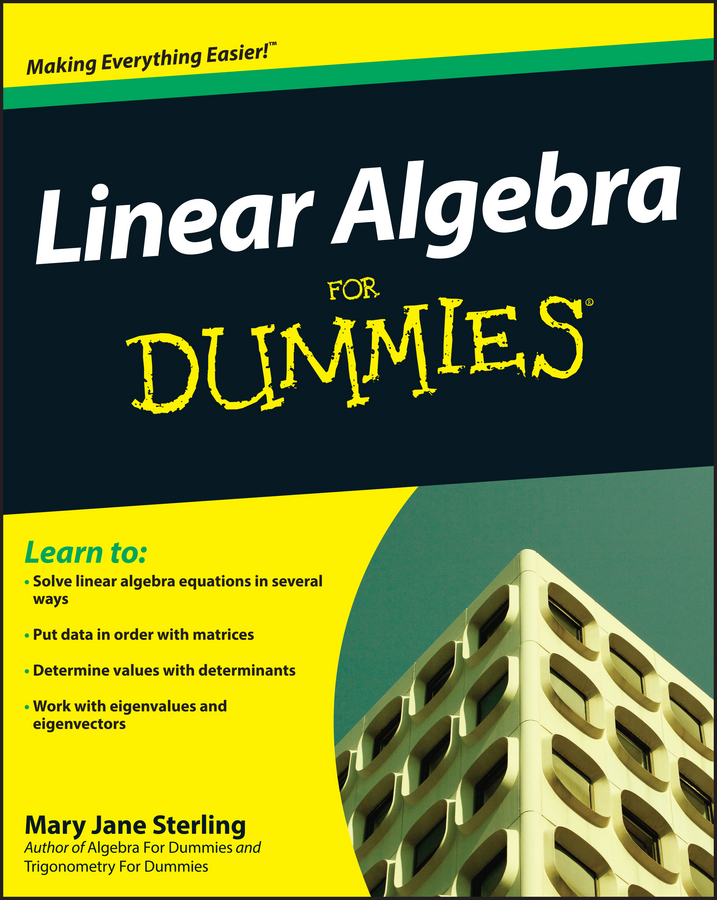A fairly new method, or algorithm, called the box method is being used to multiply two binomials together. When a trinomial of the form ax2 + bx + c can be factored into the product of two binomials, the format of the factorization is (dx + e)(fx + g) where d x f = a and e x g = c.

The box method enables you to fill in a two-by-two square to create the desired factorization. You still need to know the factors of a and c, but the box method gives you a more systematic process for determining which factors and terms to choose.

A common method for multiplying the two binomials together is called FOIL, and the factoring of the resulting trinomial is often referred to as unFOIL. In traditional algebra classes the unFOIL method was taught, having students find the factors of the coefficient, a, and the factors of the constant, c, and then filling in parentheses to get the desired product.

The following shows the factorization of 3x2 + 10x – 8 using the box method.

1. Draw a two-by-two square.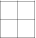2. Put the first term of the trinomial in the upper-left corner and the last term in the lower-right corner.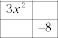3. Multiply the first and last terms: 3x2(–8) = –24x2.

4. Find two factors of the resulting product whose sum is the middle term, 10x.

Possible factors of 24x2 (these are without the negative sign) are x(24x), 2x(12x), 3x(8x), and 4x(6x).

Using 2x(12x) and making the 2x negative, you have –2x(12x) = –24x2. The sum of the two factors is –2x + 12x = 10x, the middle term of the trinomial.

5. Place the factors in the two remaining squares.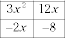6. Find the greatest common factor (GCF) of each row and each column. Write them to the side and below.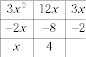7. The two entries along the right side are the terms needed in one of the binomials, and the terms along the bottom are in the other factorization. So, 3x2 + 10x – 8 = (3x – 2)(x + 4).

It doesn’t matter where you place those two factors in Step 5. If their positions had been reversed, the square would look like this: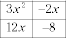And the GCFs: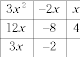You get the same entries for the binomials.